# Power BI Calculate Tutorial

Learn how to use the Power BI CALCULATE function while giving examples of how you can use it.
Aug 2022  · 6 min read

The CALCULATE function is arguably one of Power BI's most important (and most popular) DAX functions. It is easy to use and extremely versatile, allowing you to expand your data analyses and develop even more valuable Power BI reports.

This tutorial focuses on what the CALCULATE function is and how to use it and assumes you’re already familiar with the basics of Power BI and DAX.

## What is the Power BI Calculate Function?

According to the Microsoft Power BI documentation, the CALCULATE function forms part of the filter function category and is defined as "evaluating an expression in a modified filter context." An expression is essentially a measure and includes functions such as SUM, AVERAGE, and COUNT. This expression is evaluated in the context of one or more filters.

As you may know, filters can also be applied to a Power BI report simply by adding slicers without creating a measure using the CALCULATE function at all. However, there are many use cases where the CALCULATE function is more appropriate. It is especially useful to use it as a component of another function. We will see how this works in the example below for calculating the percentage of a total.

## DAX Calculate Basic Syntax

The basic DAX syntax of the CALCULATE function is:

``CALCULATE( <expression> [, <filter1> [, <filter2> [, ...]]])``

The CALCULATE function is made up of 2 key components:

• The expression - this is the aggregation component that is constructed just like a measure using functions like SUM, AVERAGE, and COUNT.
• The filters - this component allows you to specify one or more filters that control the context of the aggregation.

There are 3 types of filters that can be used in the CALCULATE function:

• Boolean filter expressions - this is a simple filter where the result must be either TRUE or FALSE.
• Table filter expressions - this is a more complex filter where the result is a table.
• Filter modification functions - filters such as ALL and KEEPFILTERS fall into this category and they give more control over the filter context you want to apply.

You can add multiple filters to the filter component of the CALCULATE function by separating each filter with a comma. All the filters are evaluated together and their order does not matter.

You can control how the filters are evaluated by using logical operators. If you want all conditions to be evaluated as TRUE then you can use AND (&&). This is also the default behavior of the filters as mentioned above. Alternatively, with the OR (||) operator, at least one condition must be evaluated as TRUE for a result to be returned.

## How to Use Power BI Calculate

To use CALCULATE, simply add a measure to your table. You can do this by navigating to the Modeling tab in the Ribbon and selecting ‘New Measure’.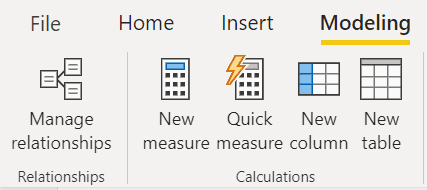Below is a simple example of the CALCULATE function using SUM to find total revenue and filtering for Country = United Kingdom. We’ll discuss this example again in more detail at the end of the tutorial.

``````UK Revenue = CALCULATE(SUM('Online Retail'[Revenue]),

'Online Retail'[Country] = "United Kingdom")``````

When viewed in a table, we can see that the UK Revenue measure is simply applying a filter for Country in addition to the filter context that is already present in the table for Month. Using the CALCULATE function in this way gives us more fine-grained control over what kind of information is displayed in our visual.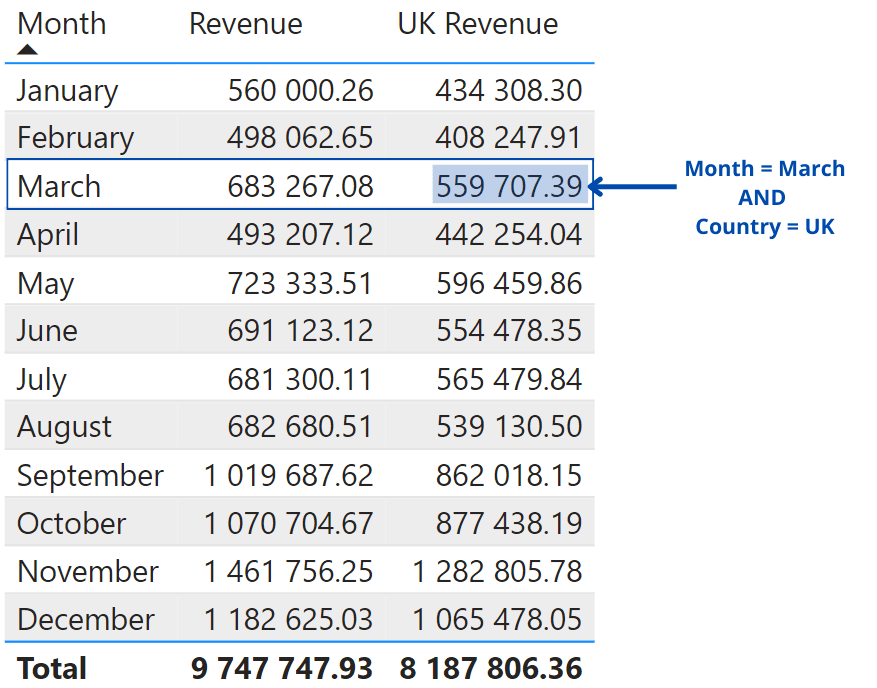An important thing to keep in mind when creating measures is to follow good data modeling practices specifically in terms of the speed and optimisation of your queries. Because of this, some uses of the CALCULATE function are faster or more appropriate than others.

For example, the Microsoft documentation recommends that you avoid using the FILTER function as an argument to other functions (such as in the CALCULATE function). Instead, it’s better to use boolean expressions where possible since they are explicitly optimized for this purpose.

In the above example we used a boolean expression to define our filter for Country = United Kingdom. This is a faster and more optimized approach. On the other hand, here is an example of the FILTER function where we get the same result but the calculation is slower:

``````UK Revenue = CALCULATE(SUM('Online Retail'[Revenue]),
FILTER('Online Retail',
'Online Retail'[Country] = "United Kingdom")``````

## Examples of Power BI Calculate Function

Using a real-world e-commerce dataset, we’ll be exploring a few key ways that the CALCULATE function can be used to solve business problems. To follow along with this tutorial, you can access the e-commerce dataset from Datacamp Workspaces.

This dataset contains information about each purchase that a customer makes: the country it was purchased from, the product description, the date and time of purchase, and the quantity and price of each product purchased.

We will be answering the following questions with the help of the CALCULATE function:

• How does the total monthly revenue in the UK compare to all other countries?
• What percentage of the total revenue is from the UK?
• What is the cumulative daily revenue?

### How does the total monthly revenue in the UK compare to all other countries?

To answer this question, we will need to create two measures using the CALCULATE function. First, we use a simple boolean filter to create a measure that returns the total revenue (using a SUM function) in the UK:

``````UK Revenue = CALCULATE(SUM('Online Retail'[Revenue]),
'Online Retail'[Country] = "United Kingdom")``````

Next, we create a similar measure but this time we use the FILTER function. This filter expression runs through each row of the Country column and returns a table containing the rows that match the filter condition. The FILTER function is required here because we are not able to return a simple TRUE or FALSE from the filter. Instead, we get a table with multiple values.

``````Non-UK Revenue = CALCULATE(SUM('Online Retail'[Revenue]),
FILTER('Online Retail',
'Online Retail'[Country] <>"United Kingdom")``````

Therefore, we can see that the UK makes up the majority of the revenue for this e-commerce store compared to all other countries.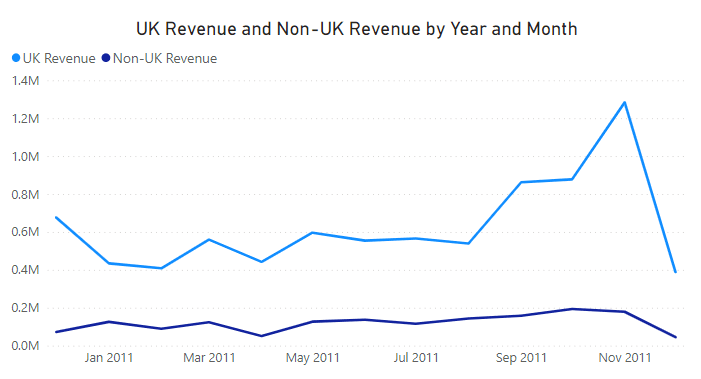### What percentage of total revenue is from the UK?

This is a very common type of question faced by Power BI developers and users and it is a perfect case to apply the CALCULATE function.

In order to find the percentage of a total, we first need to be able to return the total without it being affected by other filter contexts in the report. To achieve this we use a filter modifier known as the ALL function. Using this function, we specify which column we would like our calculation to completely ignore any filters for.

In this example, we are looking for the percentage of total revenue in the UK. This means that our calculation should ignore any filters in the Country column.

``````Total Revenue = CALCULATE(SUM('Online Retail'[Revenue]),
ALL('Online Retail'[Country]))``````

This is relevant because CALCULATE can be executed from inside a filter context that is already filtering Product[Color]. In that scenario, the presence of ALL means that the outer filter over Product[Color] is ignored and replaced with the new filter introduced by CALCULATE. This is evident if instead of slicing by Brand, we slice by Color in the matrix.

Now that we know our total revenue, we can construct a measure to show the percentage of total revenue. However, since we are specifically interested in the UK, we will use the CALCULATE function once again but this time we will use a simple boolean filter.

``````UK % of Revenue = CALCULATE(SUM('Online Retail'[Revenue])/[Total Revenue],
'Online Retail'[Country] = "United Kingdom")``````

Now we can see that the UK makes up 84% of the total revenue for this e-commerce store.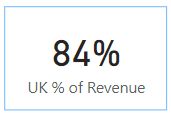### What is the cumulative daily revenue?

The cumulative revenue can give some insight into the revenue trends. By plotting this cumulative revenue on a chart, we can also visually see if revenue has increased at a faster rate or not over time.

To answer this question, we must create a measure using the CALCULATE function as well as these filter functions: ALLSELECTED, FILTER, and an evaluation using the MAX function.

``````Cumulative Revenue = CALCULATE(SUM('Online Retail'[Revenue]),
FILTER( ALLSELECTED('Online Retail'[InvoiceDate]),
'Online Retail'[InvoiceDate] <= MAX('Online Retail'[InvoiceDate])))``````

Let’s go over why these filters are important here:

• The FILTER function allows each of the 2 filters we specify to be evaluated on a row-by-row basis and will return a table in the cases where there is a match.
• The ALLSELECTED function resets the filter on InvoiceDate in the current query (in the result below the current query is the line chart) while still allowing external filters (such as from slicers).
• The MAX function is used as part of an evaluation - we want to sum the revenue for all dates that are at or below the current date in the query.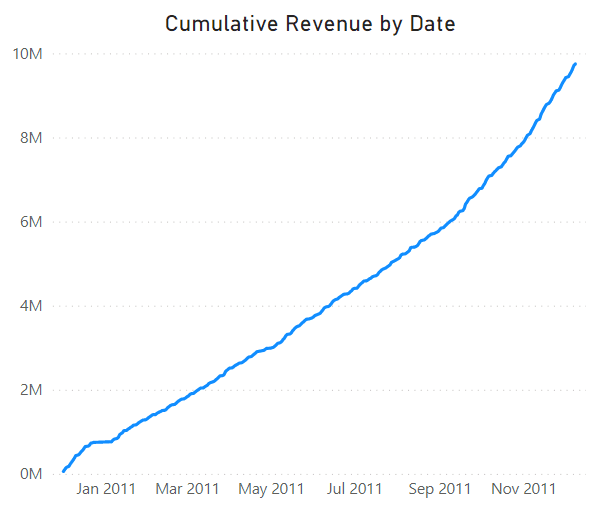## Closing Remarks

In this tutorial, we discussed what the CALCULATE function is in Power BI and how to use it. We also applied the CALCULATE function to a real-world e-commerce dataset and used it to answer some key business questions.

CALCULATE is one of the most useful functions in Power BI and you will likely need to use it frequently when building reports and generating deeper insights into your data.

Hopefully, this tutorial helps you not only understand the basic syntax of CALCULATE but also how it relates to solving real business problems as a Power BI data analyst

Courses for Power Bi

### .css-1531qan{-webkit-text-decoration:none;text-decoration:none;color:inherit;}Introduction to Power BI

Beginner
3 hr
232.4K
Master the Power BI basics and learn to use the data visualization software to build impactful reports.
See Details

### Introduction to DAX in Power BI

Beginner
2 hr
30.9K
Enhance your Power BI knowledge, by learning the fundamentals of Data Analysis Expressions (DAX) such as calculated columns, tables, and measures.

### Data Visualization in Power BI

Beginner
3 hr
26.3K
Power BI is a powerful data visualization tool that can be used in reports and dashboards.
See More
Related

### Building Your Data Science Portfolio with DataCamp Workspace (Part 1)

Learn how to build a comprehensive data science portfolio by exploring examples different examples, mastering tips to make your work stand out, and utilizing the DataCamp Workspace effectively to showcase your results.9 min### [Radar Recap] Unleashing the Power of Data Teams in 2023

Vijay Yadav and Vanessa Gonzalez will outline the keys to building high-impact data teams in 2023.### The Past, Present, and Future, of the Data Science Notebook

Jodie Burchell discusses notebooks and the challenges facing data science today.### Building a Safer Internet with Data Science

Learn the key drivers of a data strategy that helps ensure online safety and consumer protection with Richard Davis, the Chief Data Officer at Ofcom, the UK’s government-approved regulatory and competition authority.

### Conda Cheat Sheet

In this cheat sheet, learn all about the basics of working with Conda. From managing and installing packages, to working with channels & environments, learn the fundamentals of the conda package management tool suite.

### Visualizing Data in Excel